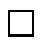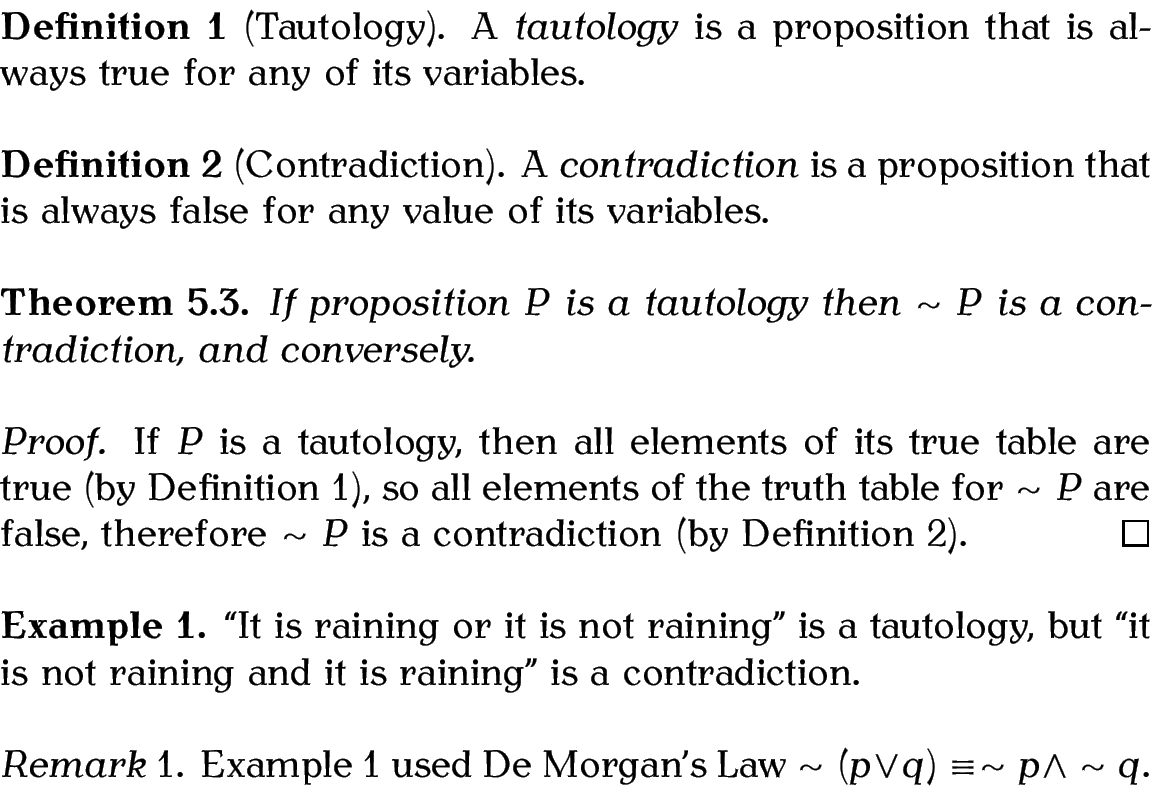## 4.7.1 The amsthm Package

The amsthm package provides three predefined theorem styles: plain, definition and remark. When you define a new theorem-like environment with \newtheorem, it is given the style currently in effect. You can change the current style with:

\theoremstyle{<style name>}

where <style name> is the name of the theorem style.

Example:

This example defines six theorem-like environments: theorem, lemma, defn, conj, note and remark. The note environment is unnumbered as it's defined using the starred version of \newtheorem. The definitions have been arranged according to the required theorem style.

The amsthm package also provides the proof environment, which can be used for typesetting proofs.

\begin{proof}[<title>]

The optional argument <title> is a replacement for the default title. This environment automatically inserts a QED symbol at the end of it, but if the default location isn't appropriate (which can happen if the proof ends with an equation) then use

where you want the QED symbol to appear. The symbol is given by

This defaults to an unfilled square, but you can redefine \qedsymbol to something else if you prefer. (Recall redefining commands from Volume 1.)

% in the preamble:

\usepackage{amsthm}
\theoremstyle{plain}
\newtheorem{theorem}{Theorem}

\theoremstyle{definition}
\newtheorem{defn}{Definition}
\newtheorem{xmpl}{Example}[chapter]

\theoremstyle{remark}
\newtheorem{remark}{Remark}

% later in the document:

\begin{defn}[Tautology]\label{def:tautology}
A \emph{tautology} is a proposition that is always true for any value of its variables.
\end{defn}

\begin{defn}[Contradiction]\label{def:contradiction}
A \emph{contradiction} is a proposition that is always false for any value of its variables.
\end{defn}

\begin{theorem}
If proposition $P$ is a tautology then $\sim P$ is a contradiction, and conversely.
\begin{proof}
If $P$ is a tautology, then all elements of its truth table are true (by Definition~\ref{def:tautology}), so all elements of the truth table for $\sim P$ are false, therefore $\sim P$ is a contradiction (by Definition~\ref{def:contradiction}).
\end{proof}
\end{theorem}

\begin{xmpl}\label{ex:rain}
It is raining or it is not raining'' is a tautology, but it is not raining and it is raining'' is a contradiction.
\end{xmpl}

\begin{remark}
Example~\ref{ex:rain} used De Morgan's Law $\sim (p \vee q) \equiv \sim p \wedge \sim q$.
\end{remark}

Result:A new theorem style can be created using

\newtheoremstyle{<name>}{<space above>}{<space below>}{<body font>}{<indent>}{<head font>}{<post head punctuation>}{<post head space>}{<head spec>}

This defines a new theorem style called <name>, which can later be set using \theoremstyle. The other arguments are as follows:

<space above>
the amount of space above the theorem-like environment
<space below>
the amount of space below the theorem-like environment
<body font>
the font to be used in the main theorem body
<indent>
the amount of indentation (empty means no indent or use parindent for normal paragraph indentation)
the font to be used in the theorem header
the punctuation to be inserted after the theorem head
the space to put after the theorem head (use {} for a normal interword space or \newline for a linebreak)

Example:

This example creates a new style called note that inserts a space of 2ex above the theorem and 2ex below.4.2 The body font is just the normal font. There is no indent, the theorem header is in small caps, a full stop is put after the theorem head and a line break is inserted between the theorem head and body:

\newtheoremstyle{note}% style name
{2ex}% above space
{2ex}% below space
{}% body font
{}% indent amount
{\scshape}% head font
{\newline}% post head punctuation

Once you have defined the style, you can now use it. For example (in the preamble):

This defines a theorem-like environment called scnote. You can now use it later in the document:

\begin{scnote}
This is an example of a theorem-like environment.
\end{scnote}

This produces:#### Footnotes

... below.4.2
Recall the ex unit from Volume 1.

This book is also available as A4 PDF or 12.8cm x 9.6cm PDF or paperback (ISBN 978-1-909440-02-9).

© 2013 Dickimaw Books. "Dickimaw", "Dickimaw Books" and the Dickimaw parrot logo are trademarks. The Dickimaw parrot was painted by Magdalene Pritchett.